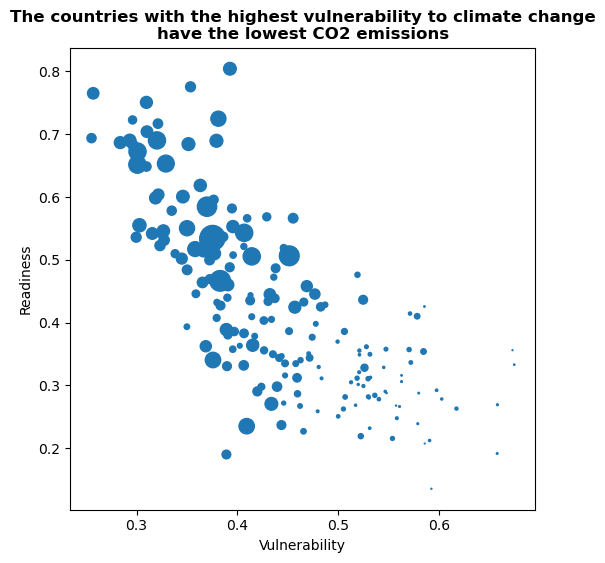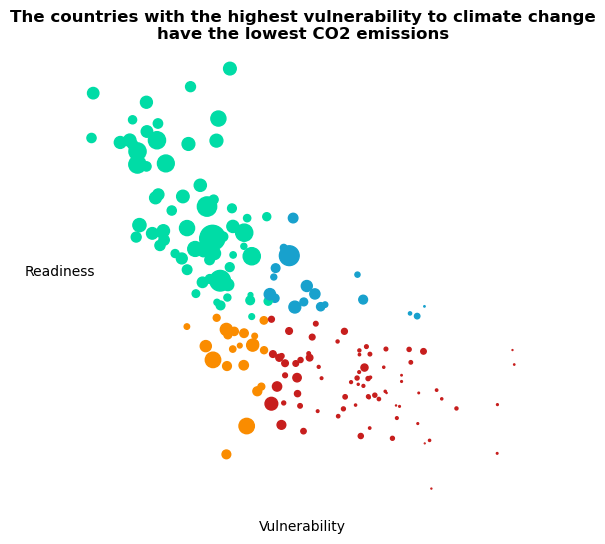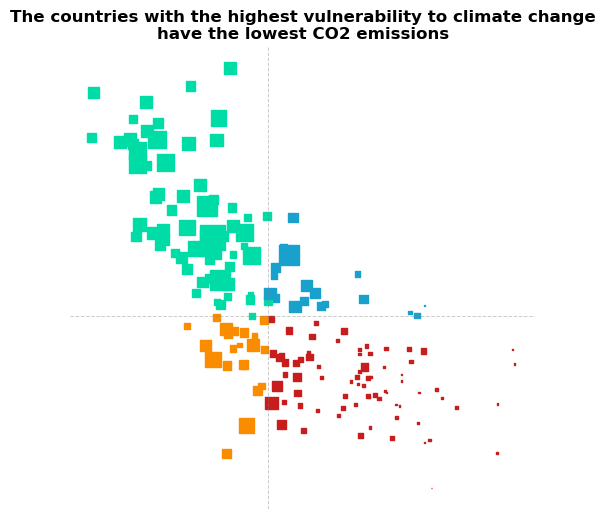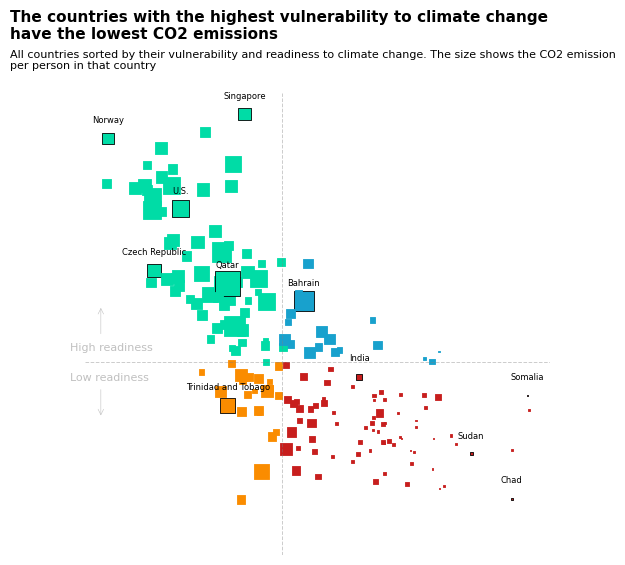This plot is a bubble plot. It shows the relation between CO2 emission, vulnerability and readiness to climate change. The size of the bubble is the CO2 emission per habitant in the country.

The chart was originally made with React. This post is a translation to Python by Joseph B..

Thanks to him for accepting sharing his work here!

Let's see what the final picture will look like:

IMAGE

## Libraries

First, you need to install the following librairies:

• matplotlib is used for creating the chart and add customization features
• `pandas` is used to put the data into a dataframe

And that's it!

``````# Libraries
import matplotlib.pyplot as plt
import pandas as pd``````

## Dataset

For this reproduction, we're going to retrieve the data directly from the gallery's Github repo. This means we just need to give the right url as an argument to pandas' `read_csv()` function to retrieve the data.

``````# Open the dataset from Github
url = "https://raw.githubusercontent.com/holtzy/the-python-graph-gallery/master/static/data/dataConsumerConfidence.csv"

# Remove the first one when merging

## Bubble plot with default layout

We'll start by creating a "simple" graph, with little customization in order to be progressive. We will create a basic bubble plot, which is just a more sophisticated scatter plot.

``````# Init the figure and axe
fig, ax = plt.subplots(figsize=(6, 6))

# Create the plot
ax.scatter(df['&nbsp;'], # x-axis
df['&nbsp;.1'], # y-axis
s=df['CO2 per Capita']*10, # size of the bubble (put on a higher scale with *10)
)

ax.set_title('The countries with the highest vulnerability to climate change\nhave the lowest CO2 emissions',
weight='bold')
ax.set_xlabel('Vulnerability')

# Show the plot
plt.show()``````## Custom color and improve layout

In order to change the color, we just have to add to the `c` argument with the name of the column containing the colors, which is `'Color'` in our case.

Then, we remove the spines and ticks from the chart in order to make it nicer

``````# Init the figure and axe
fig, ax = plt.subplots(figsize=(6, 6))

# Create the plot
ax.scatter(df['&nbsp;'], # x-axis
df['&nbsp;.1'], # y-axis
s=df['CO2 per Capita']*10, # size of the bubble (put on a higher scale with *10)
c=df['Color'],
)

ax.set_title('The countries with the highest vulnerability to climate change\nhave the lowest CO2 emissions',
weight='bold',)
ax.set_xlabel('Vulnerability')
rotation=0, # shift it horizontally
)

# Remove the spines (border lines) and scale from the chart
ax.spines['top'].set_visible(False)
ax.spines['right'].set_visible(False)
ax.spines['bottom'].set_visible(False)
ax.spines['left'].set_visible(False)
ax.set_xticklabels([])
ax.set_yticklabels([])
ax.tick_params(axis='both', which='both', length=0)

# Show the plot
plt.show()``````## Update markers type and add reference lines

To respect the original work, we're now going to change the style of the markers to square and add reference lines in the middle of the graphic.

• change the marker type: put `marker='s'` when calling the `scatter()` function
• add reference lines: use the `axvline()` and `axhline()` functions and specify the relative position, color, style, width and opacity
``````# Init the figure and axe
fig, ax = plt.subplots(figsize=(6, 6))

# Create the plot
ax.scatter(df['&nbsp;'], # x-axis
df['&nbsp;.1'], # y-axis
s=df['CO2 per Capita']*10, # size of the bubble (put on a higher scale with *10)
c=df['Color'],
marker='s'
)

ax.set_title('The countries with the highest vulnerability to climate change\nhave the lowest CO2 emissions',
weight='bold')

ax.axvline(0.43, color='gray', linestyle='--', linewidth=0.7, alpha=0.4)
ax.axhline(0.41, color='gray', linestyle='--', linewidth=0.7, alpha=0.4)

# Remove the spines (border lines) and scale from the chart
ax.spines['top'].set_visible(False)
ax.spines['right'].set_visible(False)
ax.spines['bottom'].set_visible(False)
ax.spines['left'].set_visible(False)
ax.set_xticklabels([])
ax.set_yticklabels([])
ax.tick_params(axis='both', which='both', length=0)

# Show the plot
plt.show()``````The chart is starting to look really nice! It's time to add the annotations and finish it!

## Circle some countries and add annotations

The most complex part of this section is to automate the process of surrounding a country.

To do this, we create a `circle_countries()` function that takes as argument a list of country names and returns the associated list of border colors (`'black'` for the countries in the list, `default` otherwise).

``````def circle_countries(country_names: list):

# Init the edge color parameter with its default value: same as font color
df['EdgeColor'] = df['Color']

# Change the edge color of the countries in the list
df.loc[df['Name'].isin(country_names), 'EdgeColor'] = 'black'

return df['EdgeColor']``````

Then we create a `add_country_name()` function that will add the name of the country on top of its marker. Our function will iterate over each name in the country names list, find their position on the chart using `loc()` function and add their name at this position thanks to the `text()` function.

``````def add_country_name(country_names: list):

# Iterate over each country name
for country_name in country_names:

# Find position of the country on the axes
x_axis = df.loc[df['Name']==country_name, '&nbsp;']
y_axis = df.loc[df['Name']==country_name, '&nbsp;.1']

# Add the text at the right position, slighly shift to the top for lisibility
ax.text(x_axis, y_axis+0.025, # position
country_name, # label
size=6, # size of the text
ha='center', # align the text
)``````

Then, we add the last annotations such as the `labels`, `title` and the `arrows` using `text()` and `annotate()` functions.

``````# Init the figure and axe
fig, ax = plt.subplots(figsize=(6, 6))

# Define country to circle
country_to_circle = ['Norway', 'Singapore', 'U.S.', 'Czech Republic', 'Qatar', 'Bahrain',

# Define the edgecolors according to the list
edgecolors = circle_countries(country_to_circle)

# Create the plot
ax.scatter(df['&nbsp;'], # x-axis
df['&nbsp;.1'], # y-axis
s=df['CO2 per Capita']*10, # size of the bubble (put on a higher scale with *10)
c=df['Color'],
edgecolor=edgecolors,
linewidths=0.6,
marker='s',
zorder=2
)

# Add country names on top on each marker

title = 'The countries with the highest vulnerability to climate change\nhave the lowest CO2 emissions'
fig.text(0, 0.97, # relative postion
title,
fontsize=11, # High font size for style
ha='left', # align to the left
family='dejavu sans',
weight='bold'
)

subtitle = 'All countries sorted by their vulnerability and readiness to climate change. The size shows the CO2 emission\nper person in that country'
fig.text(0, 0.92, # relative postion
subtitle,
fontsize=8, # High font size for style
ha='left', # align to the left
family='dejavu sans',
multialignment='left'
)

ax.axvline(0.43, color='gray', linestyle='--', linewidth=0.7, alpha=0.4)
ax.axhline(0.41, color='gray', linestyle='--', linewidth=0.7, alpha=0.4)

# Remove the spines (border lines) and scale from the chart
ax.spines['top'].set_visible(False)
ax.spines['right'].set_visible(False)
ax.spines['bottom'].set_visible(False)
ax.spines['left'].set_visible(False)
ax.set_xticklabels([])
ax.set_yticklabels([])
ax.tick_params(axis='both', which='both', length=0)

fig.text(0.1,0.45,
color='silver',
size=8)
fig.text(0.1,0.4,
color='silver',
size=8)

arrowprops = dict(arrowstyle="->", color='silver', lw=0.4)
ax.annotate("", xy=(0.25, 0.32), xytext=(0.25, 0.37),
arrowprops=arrowprops)
ax.annotate("", xy=(0.25, 0.5), xytext=(0.25, 0.45),
arrowprops=arrowprops)

# Show the plot
plt.show()``````## Going further

This article explains how to reproduce a bubble plot with annotations, custom colors and nice features.

For more examples of advanced customization in bubble plot, check out this other very nice plot. Also, you might be interested in adding text without overlapping to your chart.

## Contact & Edit

👋 This document is a work by Yan Holtz. You can contribute on github, send me a feedback on twitter or subscribe to the newsletter to know when new examples are published! 🔥